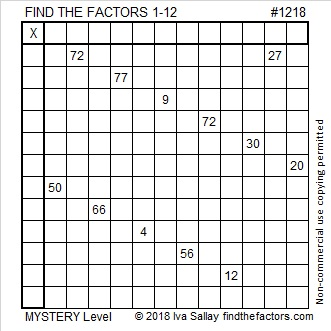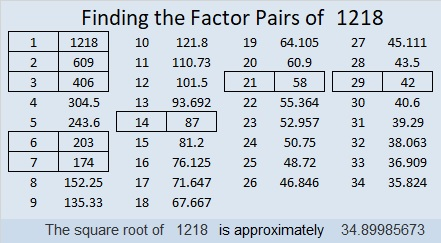# 1218 Mystery Level

Working on this Mystery level puzzle may seem easy at first, but will it remain easy until its unique solution is found? Only those who solve it will know the answer to that question!Print the puzzles or type the solution in this excel file: 12 factors 1211-1220

Here are some facts about the number 1218:

• 1218 is a composite number.
• Prime factorization: 1218 = 2 × 3 × 7 × 29
• The exponents in the prime factorization are 1, 1, 1, and 1. Adding one to each and multiplying we get (1 + 1)(1 + 1)(1 + 1)(1 + 1) = 2 × 2 × 2 × 2 = 16. Therefore 1218 has exactly 16 factors.
• Factors of 1218: 1, 2, 3, 6, 7, 14, 21, 29, 42, 58, 87, 174, 203, 406, 609,
• 1218
• Factor pairs: 1218 = 1 × 1218, 2 × 609, 3 × 406, 6 × 203, 7 × 174, 14 × 87, 21 × 58, or 29 × 42
• 1218 has no square factors that allow its square root to be simplified. √1218 ≈ 34.899861218 is the hypotenuse of a Pythagorean triple:
840-882-1218 which is (20-21-29) times 42